×#### Thank you for registering.

One of our academic counsellors will contact you within 1 working day.

Click to Chat

1800-1023-196

+91-120-4616500

CART 0

• 0

MY CART (5)

Use Coupon: CART20 and get 20% off on all online Study Material

ITEM
DETAILS
MRP
DISCOUNT
FINAL PRICE
Total Price: Rs.

There are no items in this cart.
Continue Shopping• Complete JEE Main/Advanced Course and Test Series
• OFFERED PRICE: Rs. 15,900
• View Details

```Chapter 6: Exponents Exercise – 6.1

Question: 1

Find the values of each of the following:

(i) 132

(ii) 73

(iii) 34

Solution:

(i) 132 =  13 × 13

= 169

(ii) 73 = 7 × 7 × 7

= 343

(iii) 34 = 3 × 3 × 3 × 3

= 81

Question: 2

Find the value of each of the following:

(i) (-7)2

(ii) (-3)4

(iii)  (-5)5

Solution:

We know that if ‘a’ is a natural number, then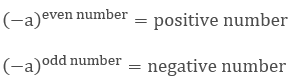We have,

(i)  (-7)2 = (-7) × (-7)

= 49

(ii) (-3)4 = (-3) × (-3) × (-3) × (-3)

= 81

(iii) (-5)5 = (-5) × (-5) × (-5) × (-5) × (-5)

= -3125

Question: 3

Simply:

(i) 3 × 102

(ii) 22 × 53

(iii) 33 × 52

Solution:

(i) 3 × 102 = 3 × 10 × 10

= 3 × 100

= 300

(ii) 22 × 53 = 2 × 2 × 5 × 5 × 5

= 4 × 125

= 500

(iii) 33 × 52 = 3 × 3 × 3 × 5 × 5

= 27 × 25

= 675

Question: 4

Simply:

(i)  32 × 104

(ii)  24 × 32

(iii) 52 × 34

Solution:

(i)  32 × 104 = 3 × 3 × 10 × 10 × 10 × 10

= 9 × 10000

= 90000

(ii) 24 × 32 = 2 × 2 × 2 × 2 × 3 × 3

= 16 × 9

= 144

(iii) 52 × 34 = 5 × 5 × 3 × 3 × 3 × 3

= 25 × 81

= 2025

Question: 5

Simply:

(i) (-2) × (-3)3

(ii) (-3)2 × (-5)3

(iii) (-2)5 × (-10)2

Solution:

(i) (-2) × (-3)3 = (-2) × (-3) × (-3) × (-3)

= (-2) × (-27)

= 54

(ii) (-3)2 × (-5)3 = (-3) × (-3) × (-5) × (-5) × (-5)

= 9 × (-125)

= -1125

(iii) (-2)5 × (-10)2 = (-2) × (-2) × (-2) × (-2) × (-2) × (-10) × (-10)

= (-32) × 100

= -3200

Question: 6

Simply:

(i) (3/4)2

(ii) (-2/3)4

(iii) (- 4/5)5

Solution: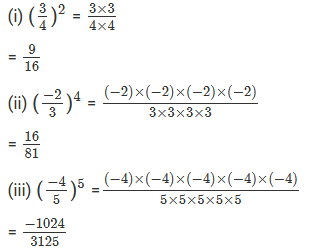Question: 7

Identify the greater number in each of the following

(i) 25 or 52

(ii) 34 or 43

(iii) 35 or 53

Solution:

(i) 25 or 52

25 = 2 × 2 × 2 × 2 × 2

= 32

52 = 5 × 5

= 25

Therefore, 25 52

(ii) 34 or 43

= 34 = 3 × 3 × 3 × 3

= 81

= 43 = 4 × 4 × 4

= 64

Therefore, 34 43

(iii) 35 or 53

= 35 = 3 × 3 × 3 × 3 × 3

= 243

= 53 = 5 × 5 × 5

= 125

Therefore, 35 53

Question: 8

Express each of the following in exponential form

(i) (-5) × (-5) × (-5)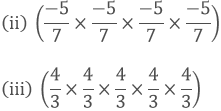Solution:

(i) (-5) × (-5) × (-5) = (-5)3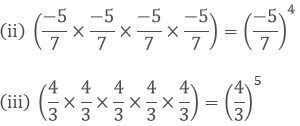Question: 9

Express each of the following in exponential form

(i) x × x × x × x × a × a × b × b × b

(ii) (-2) × (-2) × (-2) × (-2) × a × a × a

(iii) (-2/3) × (-2/3) × x × x × x

Solution:

(i) x × x × x × x × a × a × b × b × b = x4a2b3

(ii) (-2) × (-2) × (-2) × (-2) × a × a × a = (-2)4a3

(iii) (-2/3) × (-2/3) × x × x × x = (-2/3)2 x3

Question: 10

Express each of the following numbers in exponential form

(i) 512

(ii) 625

(iii) 729

Solution:

(i) 512 = 29

(iii) 625 = 54

(iii) 729 = 36

Question: 11

Express each of the following numbers as a product of powers of their prime factors

(i) 36

(ii) 675

(iii) 392

Solution:

(i) 36 = 2 × 2 × 3 × 3

= 22 × 32

(ii) 675 = 3 × 3 × 3 × 5 × 5

= 33 × 52

(iii) 392 = 2 × 2 × 2 × 7 × 7

= 23 × 72

Question: 12

Express each of the following numbers as a product of powers of their prime factors

(i) 450

(ii) 2800

(iii) 24000

Solution:

(i) 450 = 2 × 3 × 3 × 5 × 5

= 2 × 32 × 52

(ii) 2800 = 2 × 2 × 2 × 2 × 5 × 5 ×7

= 24 × 52 × 7

(iii) 24000 = 2 × 2 × 2 × 2 × 2 × 2 × 3 × 5 × 5 × 5

= 25 × 3 × 53

Question: 13

Express each of the following as a rational number of the form p/q

(i) (3/7)2

(ii) (7/9)3

(iii) (-2/3)4

Solution: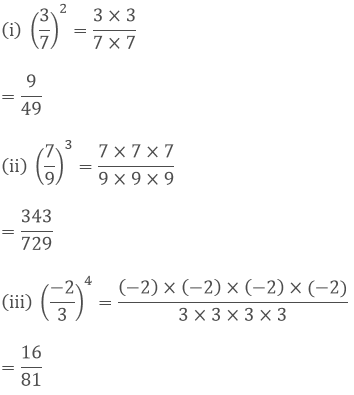Question: 14

Express each of the following rational numbers in power notation

(i) 49/64

(ii) - 64/125

(iii) -12/16

Solution:

(i) 49/64 = (7/8)2

Because 72 = 49 and 82 = 64

(ii) - 64/125 = (- 4/5)3

Because 43 = 64 and 53 = 125

(iii) – (1/216) = - (1/6)3

Because 13 = 1 and 63 = 216

Question: 15

Find the value of the following

(i) (-1/2)2 × 23 × (3/4)2

(ii) (-3/5)4 × (4/9)4 × (-15/18)2

Solution:

(i) (-1/2)2 × 23 × (3/4)2 = 1/4 × 8 × 9/16

= 9/8

(ii) (-3/5)4 × (4/9)4 × (-15/18)2 = 81/625 × 256/6561 × 225/324 = 64/18225

Question: 16

If a = 2 and b= 3, the find the values of each of the followimg

(i) (a + b)a

(ii) (ab)b

(iii) (b/a)b

(iv) (a/b + b/a)a

Solution:

(i) (a + b)a = (2 + 3)2

= (5)2

= 25

(ii) (ab)b = (2 × 3)3

= (6)3

= 216

(iii) (b/a)b = (3/2)3

= 27/8

(iv) (a/b + b/a)a = (2/3 + 3/2)2

= 169/36
```### Course Features

• 728 Video Lectures
• Revision Notes
• Previous Year Papers
• Mind Map
• Study Planner
• NCERT Solutions
• Discussion Forum
• Test paper with Video Solution